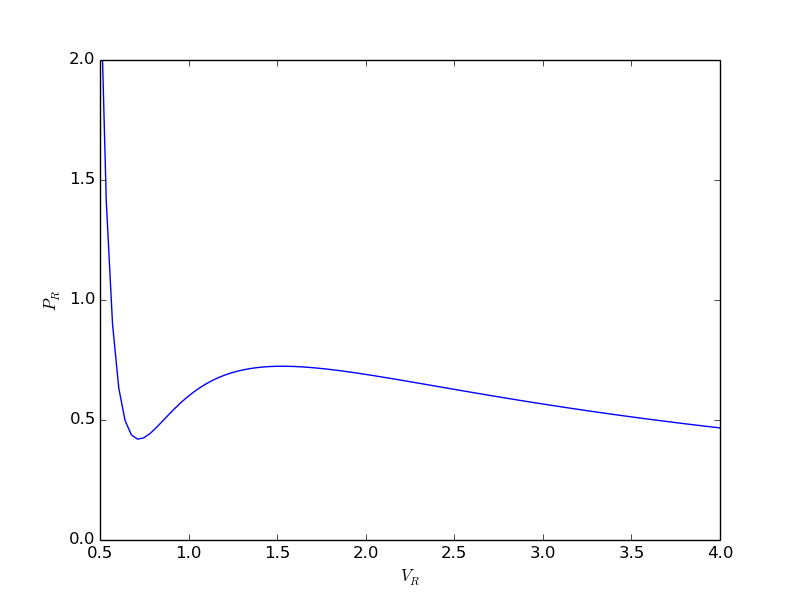The equal area method for the van der Waals equation

| categories: plotting | tags: thermodynamics | View Comments

When a gas is below its Tc the van der Waal equation oscillates. In the portion of the isotherm where $$\partial P_R/\partial V_r > 0$$, the isotherm fails to describe real materials, which phase separate into a liquid and gas in this region.

Maxwell proposed to replace this region by a flat line, where the area above and below the curves are equal. Today, we examine how to identify where that line should be.

import numpy as np
import matplotlib.pyplot as plt

Tr = 0.9 # A Tr below Tc:  Tr = T/Tc
# analytical equation for Pr. This is the reduced form of the van der Waal
# equation.
def Prfh(Vr):
return  8.0 / 3.0 * Tr / (Vr - 1.0 / 3.0) - 3.0 / (Vr**2)

Vr = np.linspace(0.5, 4, 100)  # vector of reduced volume
Pr = Prfh(Vr)                 # vector of reduced pressure

plt.plot(Vr,Pr)
plt.ylim([0, 2])
plt.xlabel('$V_R$')
plt.ylabel('$P_R$')
plt.savefig('images/maxwell-eq-area-1.png')

>>> >>> >>> >>> >>> >>> ... ... ... ... >>> >>> >>> >>> [<matplotlib.lines.Line2D object at 0x042FDAF0>]
(0, 2)
<matplotlib.text.Text object at 0x04237CB0>
<matplotlib.text.Text object at 0x042DC030>The idea is to pick a Pr and draw a line through the EOS. We want the areas between the line and EOS to be equal on each side of the middle intersection. Let us draw a line on the figure at y = 0.65.

y = 0.65

plt.plot([0.5, 4.0], [y, y], 'k--')
plt.savefig('images/maxwell-eq-area-2.png')

>>> [<matplotlib.lines.Line2D object at 0x042FDCD0>]To find the areas, we need to know where the intersection of the vdW eqn with the horizontal line. This is the same as asking what are the roots of the vdW equation at that Pr. We need all three intersections so we can integrate from the first root to the middle root, and then the middle root to the third root. We take advantage of the polynomial nature of the vdW equation, which allows us to use the roots command to get all the roots at once. The polynomial is $$V_R^3 - \frac{1}{3}(1+8 T_R/P_R) + 3/P_R - 1/P_R = 0$$. We use the coefficients t0 get the roots like this.

vdWp = [1.0, -1. / 3.0 * (1.0 + 8.0 * Tr / y), 3.0 / y, - 1.0 / y]
v = np.roots(vdWp)
v.sort()
print v

plt.plot(v, y, 'bo', v, y, 'bo', v, y, 'bo')
plt.savefig('images/maxwell-eq-area-3.png')

>>> >>> [ 0.60286812  1.09743234  2.32534056]
>>> [<matplotlib.lines.Line2D object at 0x0439C570>, <matplotlib.lines.Line2D object at 0x043933B0>, <matplotlib.lines.Line2D object at 0x04393CB0>]1 Compute areas

for A1, we need the area under the line minus the area under the vdW curve. That is the area between the curves. For A2, we want the area under the vdW curve minus the area under the line. The area under the line between root 2 and root 1 is just the width (root2 - root1)*y

from scipy.integrate import quad

A1, e1 = (v - v) * y - quad(Prfh,  v, v)
A2, e2 = quad(Prfh, v, v) - (v - v)* y

print A1, A2
print e1, e2  # interesting these look so large

>>> >>> >>> >>> 0.063225945606 0.0580212098122
0.321466743765 -0.798140339268

from scipy.optimize import fsolve

def equal_area(y):
Tr = 0.9
vdWp = [1, -1.0 / 3 * ( 1.0 + 8.0 * Tr / y), 3.0 / y,  -1.0 / y]
v = np.roots(vdWp)
v.sort()
A1 = (v - v) * y - quad(Prfh, v, v)
A2 = quad(Prfh, v, v) - (v - v) * y
return  A1 - A2

y_eq, = fsolve(equal_area, 0.65)
print y_eq

Tr = 0.9
vdWp = [1, -1.0 / 3 * ( 1.0 + 8.0 * Tr / y_eq), 3.0 / y_eq,  -1.0 / y_eq]
v = np.roots(vdWp)
v.sort()

A1, e1 = (v - v) * y_eq - quad(Prfh,  v, v)
A2, e2 = quad(Prfh, v, v) - (v - v) * y_eq

print A1, A2

>>> ... ... ... ... ... ... ... ... >>> >>> 0.646998351872
>>> >>> >>> >>> >>> >>> >>> >>> >>> 0.0617526473994 0.0617526473994


Now let us plot the equal areas and indicate them by shading.

fig = plt.gcf()

ax.plot(Vr,Pr)

hline = np.ones(Vr.size) * y_eq

ax.plot(Vr, hline)
ax.fill_between(Vr, hline, Pr, where=(Vr >= v) & (Vr <= v), facecolor='gray')
ax.fill_between(Vr, hline, Pr, where=(Vr >= v) & (Vr <= v), facecolor='gray')

plt.text(v, 1, 'A1 = {0}'.format(A1))
plt.text(v, 1, 'A2 = {0}'.format(A2))
plt.xlabel('$V_R$')
plt.ylabel('$P_R$')
plt.title('$T_R$ = 0.9')

plt.savefig('images/maxwell-eq-area-4.png')
plt.savefig('images/maxwell-eq-area-4.svg')

>>> >>> [<matplotlib.lines.Line2D object at 0x043939D0>]
>>> >>> >>> [<matplotlib.lines.Line2D object at 0x043A7230>]
>>> <matplotlib.text.Text object at 0x0438E730>
<matplotlib.text.Text object at 0x047B7930>
<matplotlib.text.Text object at 0x04237CB0>
<matplotlib.text.Text object at 0x042DC030>
<matplotlib.text.Text object at 0x042EBCD0>